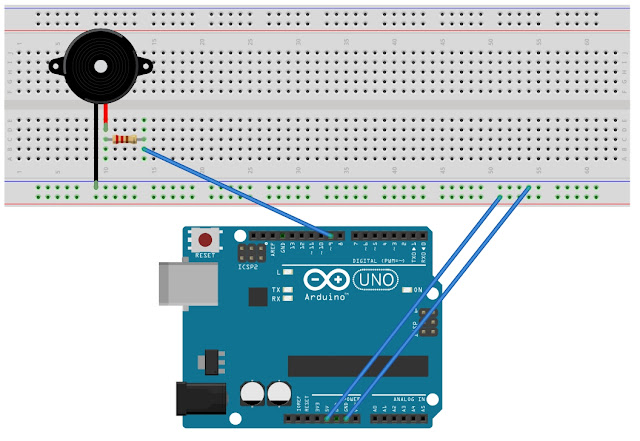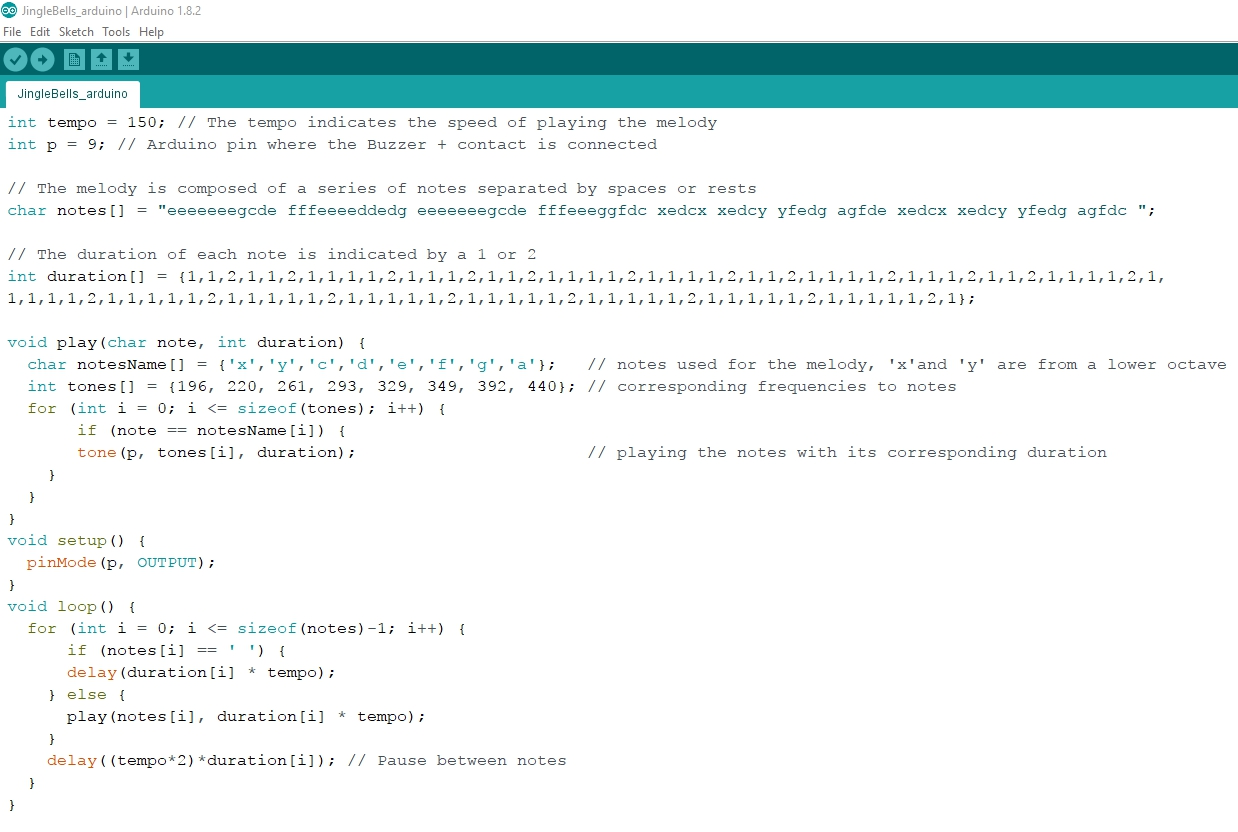## Saturday, July 1, 2017

### 2 - Arduino: piezoelectric buzzer playing Jingle Bells

ARDUINO: PIEZOELECTRIC BUZZER PLAYING JINGLE BELLS

1 - Introduction

-  A buzzer is an electromechanical component that can be used to make noise or even play music.

- Inside the buzzer there is a coil of wire and a small magnet. When current flows through the coil it becomes magnetized and pulls towards the magnet creating a tiny "click". When the click is played thousands of times per second tones are created.

- The Arduino has a built-in command called tone() which clicks the buzzer at a certain frequency.

- Frequencies used in this exercise and their corresponding notes:

x (g for a lower octave) = 196 Hz
y (a for a lower octave) = 220 Hz
c = 261 Hz
d = 293 Hz
e = 329 Hz
f = 349 Hz
g = 392 Hz
a = 440 Hz

2 - Layout schematics

- The buzzer has two pins, one positive (marked by a "+" symbol) and the other negative.

- In this example the positive pin is connected to Arduino digital pin 9 (optionally through a resistor of 330 Ohms), and the negative pin is connected to GND.3 - Running the Arduino sketch

- The code used in this Arduino sketch:- Running the code over the circuit (the resistor of the previous schematics has been removed to obtain louder sound from the buzzer):